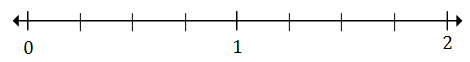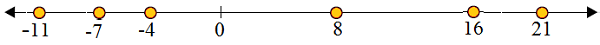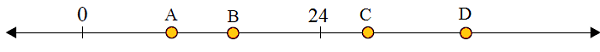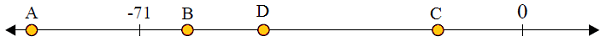# Plotting integers on a number line

#### Complete Python Prime Pack

9 Courses     2 eBooks

#### Artificial Intelligence & Machine Learning Prime Pack

6 Courses     1 eBooks

#### Java Prime Pack

9 Courses     2 eBooks

In this lesson, we learn that positive and negative numbers are used together to describe quantities having opposite values (e.g., temperature above/below zero, elevation above/below sea level, credits/debits, positive/negative electric charge). We use positive and negative numbers to represent quantities in real-world contexts, explaining the meaning of 0 in each situation.

Integers − The set of whole numbers and their opposites. are positive and negative whole numbers. These numbers do not have a fractional or decimal component.

A number line is a generally horizontal line extending infinitely in two directions shown by arrow marks. We count in each direction, in the positive direction as well as in the negative direction.First, we identify a range of values to be shown on the number line. Range is largest value minus the lowest value. We mark zero in the proper position on the number line. We then, divide the number line conveniently in equal divisions and label the divisions in jumps of 1, 2, 5 and so on. We locate the integers on the number line representing each with a colored dot.

In this lesson, we plot integers on a number line after finding the range of values given, locating the zero and labeling the divisions properly.

Plot the following integers on the number line

-11, 0, 8, -7, 16, -4, 21

### Solution

Step 1:

Range = Largest integer – Smallest integer = 21-(-11) = 21 + 11 = 32

Number line starting from -11 and ending at 21.

Step 2:

So, zero on the number line is nearer to -11 than 21. We put zero nearer to -11 about ⅓rd length to the right starting from extreme left.

Step 3:

After marking 0, -11 and 21 on the number line, we mark the remaining integers on the number line as followsWhich letter best represents 26?### Solution

Step 1:

26 lies to the right of 24 on the given number line.

Step 2:

From the image of given number line, C best represents 26 as 24 < 26.

Which letter best represents −15?### Solution

Step 1:

−15 lies to the right of −71 on the given number line.

Step 2:

From the image of given number line, C best represents −15 as −71 < −15.